# Arithmetic Sequence Explained With Nth Term & Common Difference-

Arithmetic sequence is a topic of mathematics. Often, students get confused when calculating arithmetic sequence because of the nth term and common difference.

In this post, we will learn about the basic concept of arithmetic sequence, how to find arithmetic sequence, nth term and common difference. We will also calculate arithmetic sequence with step-by-step examples. However, before moving onto the topic, let’s learn about the general concept of sequence.

## What Is A Sequence?

Sequence usually has patterns that allow us to predict what the next term might be. Ordered list of numbers is called as a sequence. Each number in a sequence is called a term.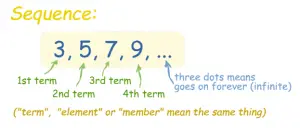### Example-01:

3, 5, 7, …

In this sequence, add 2 to get the next number.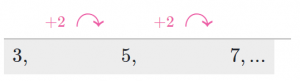Three dot means sequence moving on up to infinite numbers even through we only see a few terms e.g., the fourth term of this sequence will be nine and the fifth term will be 11 and so on.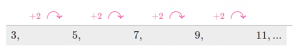### Example-02:

21, 16, 11, 6, …

In this sequence, subtract five to get the next term.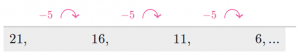Sequence term should follow the sum to get the next term or subtract, multiply, division. For example-

### Pattern

4, 11, 18, 25, … Add seven to the previous term
100, 96, 92, 88, … Subtract four the previous term
4, 12, 36, 108, … Multiply the previous term by three
4, 20, 10, 5, … Divide the previous term by two

## What Is Arithmetic Sequence?

An arithmetic sequence is a sequence in which each term increases or decreases by adding or subtracting a constant value called as common difference (d).

### OR

The pattern that involves adding or subtracting a constant number to the previous term to get next term. Sequence with such a pattern is called arithmetic sequence.

If the common difference between the terms is positive, we say that the sequence is increasing.

If the common difference between the terms is negative, we say that the sequence is decreasing.

### Example Of Increasing Sequence-

3, 5, 7, …

In this sequence, the common difference between the terms is 2 which is a positive number so the sequence is increasing.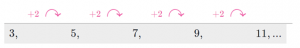### Example Of Decreasing Sequence-

-2, -5, -8, -11, …

In this sequence, the common difference between the terms is -3 which is a negative number so the sequence is decreasing.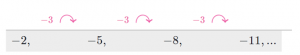## Arithmetic Sequence Formula-

If you want to find any term or the nth term of the arithmetic sequence, we use this formula of arithmetic sequence. According to this formula, we have-

an= a1 + (n-1) d

Here,

an = nth term of the sequence

a1= first term of the sequence

n = number of sequences

d = common difference between the consecutive terms

The above formula is used in Arithmetic Sequence Calculator to implement the accurate calculations of arithmetic sequence, common difference, and nth term.

## How To Find Arithmetic Sequence?

In this section, we will see examples for the calculation of arithmetic sequence, common difference, and nth term.

## Example-01:

Find the nth term and sum of arithmetic sequence of 10 number of terms if the first term is 4 and common difference is 5.

## Solution-

### Step-01:

Using the given information, we have-

a1 = 4

n = 10

d = 5

### Step-02:

Put the values in the arithmetic sequence formula. Then, we have-

an = 4 + (10-1) (5)

= 4 + 9(5)

= 4 + 45

= 49

Thus, nth term = 49.

### Step-03:

Add the common difference to the first term to get the sequence. Then, we have-

a1 = 4

a2 = a1 + d = 4 + 5 = 9

a3 = a2 + d = 9 + 5 = 14

a4 = a3 + d = 14 + 5 = 19

a5 = a4 + d = 19 + 5 = 24

a6 = a5 + d = 24 + 5 = 29

a7 = a6 + d = 29 + 5 = 34

a8 = a7 + d = 34 + 5 = 39

a9 = a8 + d = 39 + 5 = 44

a10 = a9 + d = 44 + 5 = 49

## Example-02:

Find the nth term and sum of arithmetic sequence of 12 numbers of terms if the first term is 3 and common difference is 5.

## Solution-

### Step-01:

Using the given information, we have-

a1 = 3

n = 12

d = 5

### Step-02:

Put the values in the arithmetic sequence formula. Then, we have-

an = 3 + (12-1) (5)

= 3 + 11(5)

= 3 + 55

= 58

Thus, nth term = 58.

### Step-03:

Add the common difference to the first term to get the sequence. Then, we have-

a1 = 4

a2 = a1 + d = 3 + 5 = 8

a3 = a2 + d = 8 + 5 = 13

a4 = a3 + d = 13 + 5 = 18

a5 = a4 + d = 18 + 5 = 23

a6 = a5 + d = 23 + 5 = 28

a7 = a6 + d = 28 + 5 = 33

a8 = a7 + d = 33 + 5 = 38

a9 = a8 + d = 38 + 5 = 43

a10 = a9 + d = 43 + 5 = 48

a11 = a10 + d = 48 + 5 = 53

a12 = a11 + d = 53 + 5 = 58

We can find any term of the sequence by using arithmetic sequence formula e.g., 120th term 500th term etc.

## Example-03:

Find the 120th term of arithmetic sequence if the first term is 8 and common difference is 5.

## Solution-

### Step-01:

Using the given information, we have-

a1 = 8

n = 120

d = 5

### Step-02:

Put the values in the arithmetic sequence formula. Then, we have-

an = 8 + (120-1) (5)

= 8 + 119(5)

= 8 + 595

= 603

Thus, 120th term = 603.

## Example-04:

Find the 500th term of arithmetic sequence if the first term is 9 and common difference is 7.

## Solution-

### Step-01:

Using the given information, we have-

a1 = 9

n = 500

d = 7

### Step-02:

Put the values in the arithmetic sequence formula. Then, we have-

an = 9 + (500-1) (7)

= 9 + 499(5)

= 9 + 2595

= 2604

Thus, 500th term = 2604.

Verify the above answer using the online nth Term Calculator.

## Summary-

As you have witnessed, arithmetic sequence is not that difficult or confusing to calculate. Once you have grabbed the core concept of the topic, you will easily find the sequence, nth term and common difference using the formula.

Summary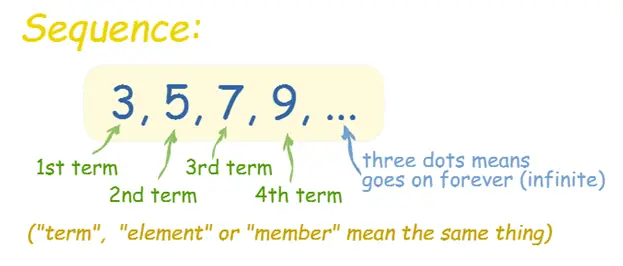Article Name
Arithmetic Sequence Explained With Nth Term & Common Difference
Description
Arithmetic Sequence Explained With Nth Term & Common Difference- Ordered list of numbers is called as a sequence. Each number in a sequence is called a term. An arithmetic sequence is a sequence in which each term increases or decreases by adding or subtracting a constant value known as common difference (d).
Author
Publisher Name
Gate Vidyalay
Publisher Logo